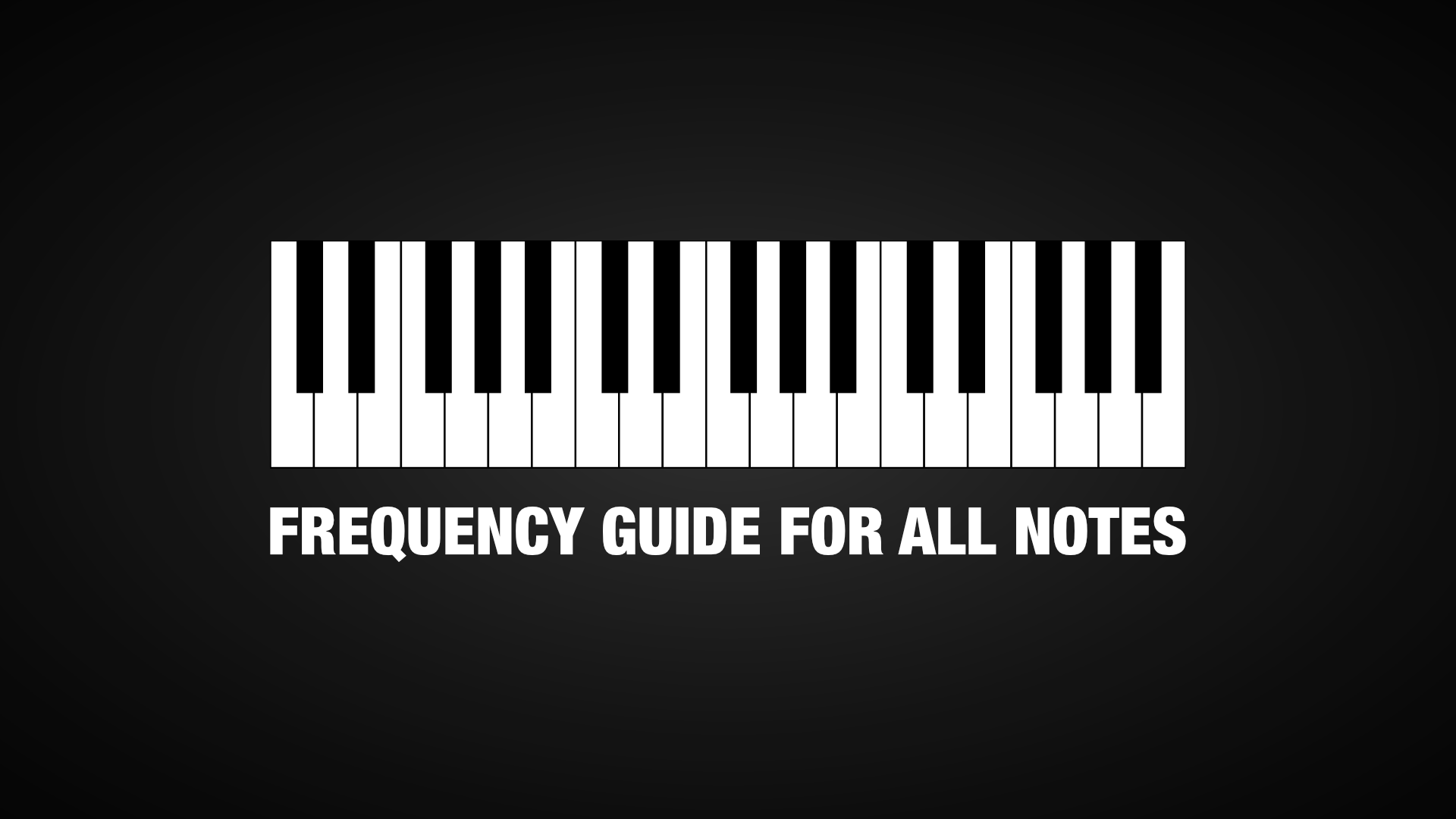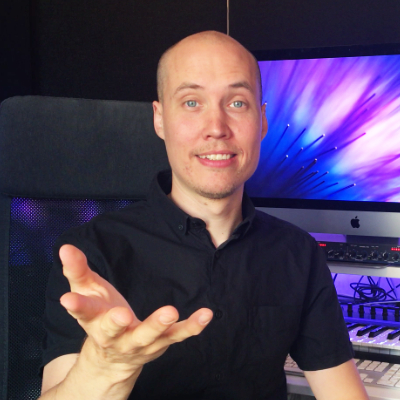Hello Music Composers, Producers and Sound Designers, Mike here! =)

I have created a Note Frequency Chart that you for example can use to perfectly tune your instruments and sounds to the key of your song, sound design etc.

The note frequency guide is based on the standard 12-tone Equal Tempered Tuning System (A = 440Hz).

# Frequency Chart for Octave 0

• C = 16.35 Hz
• C#/Db = 17.32 Hz
• D = 18.35 Hz
• D#/Eb = 19.45 Hz
• E = 20.60 Hz
• F = 21.83 Hz
• F#/Gb = 23.12 Hz
• G = 24.50 Hz
• G#/Ab = 25.96 Hz
• A = 27.50 Hz (Lowest Piano Note)
• A#/Bb = 29.14 Hz
• B = 30.87 Hz
• C = 32.70 Hz

# Frequency Chart for Octave 1

• C = 32.70 Hz
• C#/Db = 34.65 Hz
• D = 36.71 Hz
• D#/Eb = 38.89 Hz
• E = 41.20 Hz
• F = 43.65 Hz
• F#/Gb = 46.25 Hz
• G = 49.00 Hz
• G#/Ab = 51.91 Hz
• A = 55.00 Hz
• A#/Bb = 58.27 Hz
• B = 61.74 Hz

# Frequency Chart for Octave 2

• C = 65.41 Hz
• C#/Db = 69.30 Hz
• D = 73.42 Hz
• D#/Eb = 77.78 Hz
• E = 82.41 Hz
• F = 87.31 Hz
• F#/Gb = 92.50 Hz
• G = 98.00 Hz
• G#/Ab = 103.83 Hz
• A = 110.00 Hz
• A#/Bb = 116.54 Hz
• B = 123.47 Hz

# Frequency Chart for Octave 3

• C = 130.81 Hz
• C#/Db = 138.59 Hz
• D = 146.83 Hz
• D#/Eb = 155.56 Hz
• E = 164.81 Hz
• F = 174.61 Hz
• F#/Gb = 185.00 Hz
• G = 196.00 Hz
• G#/Ab = 207.65 Hz
• A = 220.00 Hz
• A#/Bb = 233.08 Hz
• B = 246.94 Hz

# Frequency Chart for Octave 4

• C = 261.63 Hz (Middle C)
• C#/Db = 277.18 Hz
• D = 293.66 Hz
• D#/Eb = 311.13 Hz
• E = 329.63 Hz
• F = 349.23 Hz
• F#/Gb = 369.99 Hz
• G = 392.00 Hz
• G#/Ab = 415.30 Hz
• A = 440.00 Hz
• A#/Bb = 466.16 Hz
• B = 493.88 Hz

# Frequency Chart for Octave 5

• C = 523.25 Hz
• C#/Db = 554.37 Hz
• D = 587.33 Hz
• D#/Eb = 622.25 Hz
• E = 659.25 Hz
• F = 698.46 Hz
• F#/Gb = 739.99 Hz
• G = 783.99 Hz
• G#/Ab = 830.61 Hz
• A = 880.00 Hz
• A#/Bb = 932.33 Hz
• B = 987.77 Hz

# Frequency Chart for Octave 6

• C = 1046.50 Hz
• C#/Db = 1108.73 Hz
• D = 1174.66 Hz
• D#/Eb = 1244.51 Hz
• E = 1318.51 Hz
• F = 1396.91 Hz
• F#/Gb = 1479.98 Hz
• G = 1567.98 Hz
• G#/Ab = 1661.22 Hz
• A = 1760.00 Hz
• A#/Bb = 1864.66 Hz
• B = 1975.53 Hz

# Frequency Chart for Octave 7

• C = 2093.00 Hz
• C#/Db = 2217.46 Hz
• D = 2349.32 Hz
• D#/Eb = 2489.02 Hz
• E = 2637.02 Hz
• F = 2793.83 Hz
• F#/Gb = 2959.96 Hz
• G = 3135.96 Hz
• G#/Ab = 3322.44 Hz
• A = 3520.00 Hz
• A#/Bb = 3729.31 Hz
• B = 3951.07 Hz

# Frequency Chart for Octave 8

• C = 4186.01 Hz (Highest Piano Note)
• C#/Db = 4434.92 Hz
• D = 4698.63 Hz
• D#/Eb = 4978.03 Hz
• E = 5274.04 Hz
• F = 5587.65 Hz
• F#/Gb = 5919.91 Hz
• G = 6271.93 Hz
• G#/Ab = 6644.88 Hz
• A = 7040.00 Hz
• A#/Bb = 7458.62 Hz
• B = 7902.13 HzMy name is Mikael “Mike” Baggström, and I am a composer, sound designer, artist and educator.

PS. Do you want to LEVEL UP as a Composer? – Learn How here.